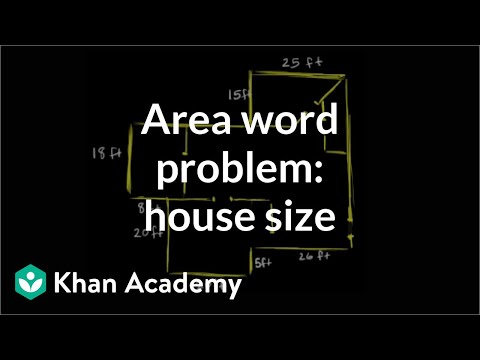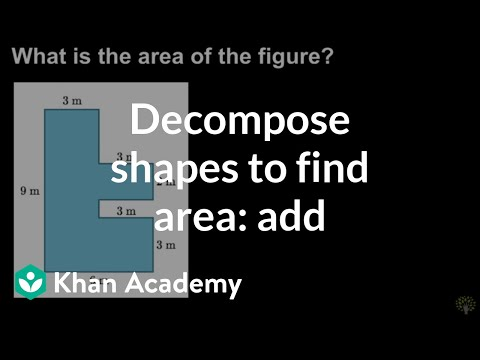Video

# Decomposing shapes to find area: subtract (Full video)

Description: Lindsay finds the area of an irregular shape by decomposing it into 2 rectangles and subtracting the area of the rectangles. So down here we have this green shaded figure, and it looks like a rectangle except it has a square cut out in the middle. It covers this rectangle's amount of area with this square cut out. So what we can do is find the area of the larger rectangle and then cut out or subtract the area of the square to see what's left in this shaded area.

### Other videos you might be interested in### Area word problem: house size (Full video)